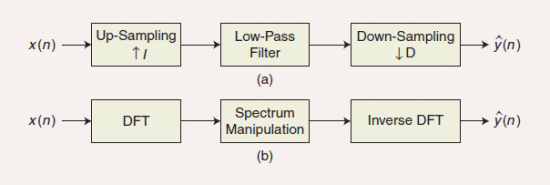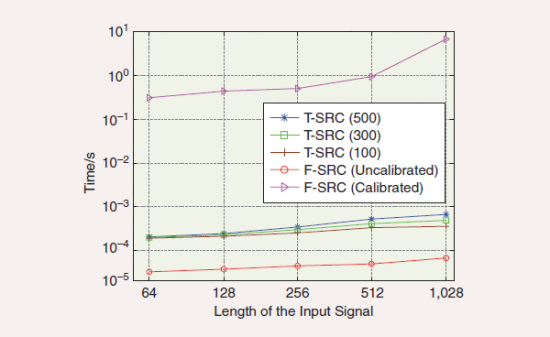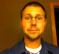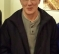## Need Help In Interpreting Curves in a ChartStarted by 6 years ago6 replieslatest reply 6 years ago158 views
In the most recent edition of the IEEE Sig. Proc. Magazine was an article discussing a new technique to perform sample rate change (SRC) . The authors started out by presenting the following Figure 1 illustrating both the time-domain and frequency-domain methods for implementing a sample rate change on the input x(n) sequence.Figure 1. The steps of SRC: (a) The time-domain method and (b) The frequency-domain method.

The topic of the article was the authors' "new, improved, and more accurate" algorithm to implement the center block in Figure 1(b) using a scheme they called "Calibrated frequency-domain SRC."  At the end of the article they presented the following Figure 5 showing the computational speed of their new "calibrated SRC" scheme compared to the computational speed of other SRC methods, for both decimation and interpolation, as implemented on a PC-compatible desktop computer.

The Legend in Figure 5 is interpreted as follows:

T-SRC (500) = SRC in Figure 1(a) using a 500-tap FIR filter.

T-SRC (300) = SRC in Figure 1(a) using a 300-tap FIR filter.

T-SRC (100) = SRC in Figure 1(a) using a 100-tap FIR filter.

F-SRC (Uncalibrated) = Figure 1(b) using a previously-published technique.

F-SRC (Calibrated) = Figure 1(b) using their proposed SRC method.Figure 5. The computation time for time-domain SRC (T-SRC), uncalibrated, and calibrated frequency-domain SRC (F-SRC). (The x-axis are the lengths of various x(n) input sequences.)

Here are my questions: How do we interpret the y-axis label, "Time/s", in Figure 5?  Do you think the "Time/s" nomenclature simply means "time measured in seconds"?  If it does then Figure 5 seems to indicate that the authors' "F-SRC (Calibrated)" method takes 10,000 times longer to compute than a previously-published "F-SRC (Uncalibrated)" method!  That doesn't seem "reasonable" to me.

Another question: For decimation, if an input sequence is 64-samples in length what does it mean to pass a 64-sample x(n) sequence through a 500-tap FIR filter prior to downsampling?  Would the filter's output sequence have any meaning?  I look forward to any opinions from you guys here on dsprelated.com?

 L. Zhao, et al, "Autocalibrated Sampling Rate Conversion in the Frequency Domain", IEEE Signal Processing Magazine, May 2017, pp. 101–106.
[ - ]It is time, in the paper they note that the "calibrated" requires more computation time but it increases performance (i.e. decreases MSE error - "significantly minimize conversion errors").  They state computational complexity for the calibrated is $$N^3$$ whereas the uncalibrated is $$NlogN$$.

[ - ]Hi Chris.  So you're saying the y-axis in Figure 5 is simply time (seconds). OK. I'll buy that.  Yes, I saw the authors' words that "the computational complexity for the calibrated scheme is N^3 whereas the complexity of the uncalibrated scheme is N*(log base 10 of N).  When I compute those two complexity values for N = 64 I see a difference by a factor of 2,267 and not 10,000 as indicated in their Figure 5.  Oh well, I won't worry about that discrepancy.

My guess is that the proposed 'calibrated freq-domain SRC' scheme in not more accurate than traditional time-domain SRC schemes that use high-performance FIR filters. But(!), the 'calibrated freq-domain SRC' scheme is terribly more computationally intensive than an equivalent-perfomance time-domain SRC scheme. To me, the authors' method is not at all useful.  Of course, I may be wrong.

[ - ]Rick,

That is a good point, I did not look at the full analysis in the article or the previous articles just looked up the plots after you posted the question.  In table 1 they state their method (calibrated) has the least error - I haven't reviewed their method to see if their approach is reasonable but it is the claim they make.

Regards,
Chris

[ - ]On the first point, I feel like "time/s" would be a measure of CPU efficiency.  As in the amount of time spent executing the algorithm every second, where the capacity of the processor is 1s/s.  Why the uncalibrated result is so poor, maybe to highlight how smart their new IP is

I expected the x axis to represent the sample rate ratio from the higher to lower rate, but maybe it's like hop size in spectral analysis?

[ - ]